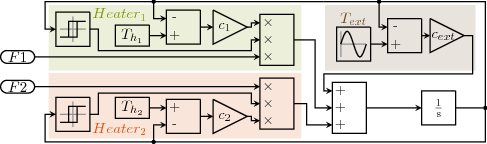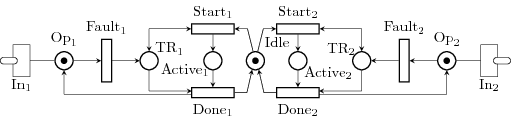###### Overview

It is easy to generate and use a C code for discrete Simulink models (using only discrete blocks, which are sampled at fixed intervals) using MathWorks tools. However, it limits the expressivity of the models. In order to use more diverse Simulink models and control the flow of a multi-model simulation (with Discrete Event Stochastic Processes) we developed a Simulink Simulation Engine embedded into Cosmos.

Like MATLAB, it computes approximate values for the signals of the model. The Cosmos' Simulink Simulation Engine only supports subset of the Simulink blocks, however large enough to show the main features of Simulink models : threshold crossings (through Switch or Relay (hysteresis) blocks), adaptative Integration methods (through Integrator blocks), latencies (through (Unit) Delay (discrete) and Transport Delay (continuous) blocks).

###### Sketch of the Cosmos Simulink Simulation loop

We first define a Block Ordering that reflects the dependencies on the computation of signals on a single simulation step. This order is defined as follows: (a.) blocks with no input signals, (b.) blocks with non-infitesimal latency by increasing latency, (c.) infinitesimal latency blocks (Integrators) and (d.) immediate blocks in topological order. Note that if no such topological order exists, there is an immediate dependency loop in the model and it cannot be simulated.

Values of signals will be stored into extensible arrays Bk,o for each output o of each block B of the model. Values of time are stored into an extensible array T. Those are initialized with the (given) initial time tinit, then the values of the blocks are generated using the block ordering. For latencied blocks, we use their initial values.

For each step, we then compute the next simulation time, through sampling times of discrete blocks. It is then refined to respect the precision of the adaptative integration method. Then, if the threshold value of any conditional block is crossed, we compute the threshold crossing time. Once this simulation time is computed, we can then compute the value of every signals processing blocks through the block ordering.

This simulation loop is more thouroughly explained in Integrating Simulink into Cosmos (to appear)

An exact semantics of Simulink models has also been defined, based on the construction of differential equations through exploration of backwards graphs of Integrator blocks, choice of modes for each conditional block, and instanciation of latency functions which allow to use past behaviour of signals to represent current behaviour of output signals of latency blocks. This allows to check whether results of the simulation engine are approximately correct or not.

Exact semantics of Simulink models are more detailed in Integrating Simulink into Cosmos (to appear)

###### Running a Simulink model with Cosmos

First, make sure you have an updated version of the OCaml compiler (at least 4.04) with the following OCaml packages: zip, xml-light and str. You also need to have compiled Cosmos. Once these requirements are installed, build the lastest version of Cosmos' Simulink Simulation Engine using, from the base folder of Cosmos sources : ```cd modelConvert/``` then `./build.sh`.

Make sure (a.) your model is registered into the .slx format and (b.) the initial simulation time is correctly registered; if necessary, convert the model into this format using Matlab-Simulink.

Once these steps are complete, to simulate a Simulink model using Cosmos, you need to (a.) compile it into Cosmos-compatible code using ```CosmosSimulink model.slx```. It should return a minimal TikZ code that represents the Simulink model, without any error. If a block is not recognized, try either modifying the Simulink model using supported blocks, or contacting the current developer (Yann Duplouy).

Finally, copy a `main.cpp` file from any of the Simulink Examples (in `Examples/Simulink`). You can now call Cosmos:

• using the interactive mode to monitor the behaviour of the model at each time step, using `Cosmos SKModel.grml -i`; then use `s` to progress by a single simulation step, `w t` to compute the model until the time t, and `q` to interrupt the simulation.
• or to process a graph of the simulation, ```Cosmos SKModel.grml --output-trace trace.data 0 --gnuplot-driver svg --max-run 1 --loop t``` where t is the final simulation time.

###### Switch, Integrators and DelayApplication of exact semantics. We simulate this model over the time interval [0,2]. Given the constant zero function for the initial step of the Transport Delay block (B3), and the intial conditions of the Integrator blocks, we know that y(0) = 1 and z(0) = 0.
Over the time interval [0,1], we are still in the initial condition of B3, hence z' = 0 which implies z(t) = 0. The condition of B1 isn't met, which implies y' = 1 hence y(t) = t.
The solution over [0,1] implies that z'(t) = t-1 on the interval [1,2], which implies, knowing z(1)=0, that z(t) = (t-1)2/2. The condition of B1 is now met, which implies y'(t) = z(t) = (t-1)2/2 and finally y(t) = (t-1)3/6+1 (because we had y(1)=1).

### Running a Petri net and a Simulink model altogether

###### Overview

We have implemented a co-simulation loop between slightly enhanced Petri nets and Simulink models into Cosmos. More specifically, we have added two kinds of transitions to Petri nets, which either send new values to the Simulink model or read Simulink signals to generate new bindings for the Petri net.

###### Example: Double Heater

This example is available inside the source code of Cosmos, under the folder `Examples/MultiModel/DoubleThermostat`. It will be soonly available on this website in a separate download.

Note: you might currently have to run `./build.sh` in the `modelConvert` folder of the `Cosmos` repository (or location) to have `CosmosSimulink` properly built and installed before running this exampleThe Simulink model modelises the temperature of a room which is heated by a system of two heaters, each having a different heat diffusion constant ci and a own temperature of Ti. It also modelises the interaction with the external temperature. The model has two inputs, each representing whether a heater is working correctly or out of order.

The two Relay block (with a symbol of hysteresis) controls whether the heaters should be working. In this model, we try to keep the temperature between 20°C and 25°C.The Petri net both controls the failure rate of each of the heater (with an exponential distribution) and also the behavior of a repairman, which chooses and repair an heater in both a fixed time.

The synchronisation is done through the transitions In1 and In2 which are both synchronisation transitions. They copy the value of Opi (here, a number of tokens) to the signals Hi of the Simulink model.

Note that every synchronisation procedure is encoded into the GSPN model. In the `benchmarkPN18.grml` model given in this example, we also read the temperature given by the Simulink model, through the `Synch21O1` transition, connected to the temperature signal.

Running the examples. One can replicate the results presented in the Integrating Simulink into Cosmos article using both `benchmarkPN18.sh` and `benchmarkPN18_dti.sh` shell scripts. They both consists of two instructions:

• first, compile the Simulink model using `CosmosSimulink`. Several options are given. The first, `--epsv 0.01` is for the precision step of the ODE45 procedure for the Integrator blocks. The second, `--skbasestep 1` gives the base step (before Integration and Threshold Crossing) of the Simulink model. Finally, the model file is given.
`CosmosSimulink --epsv 0.01 --skbasestep 1 benchmarkPN18.slx`
• second, start Cosmos using first the GRML then the HASL formula you want to check. Here, we used `--njob 6` so Cosmos runs 6 simulations in parallel, and `--max-run 500000` to precise the number of simulations we want to run.
`Cosmos benchmarkPN18.grml benchmarkPN18.lha --njob 6 --max-run 500000`